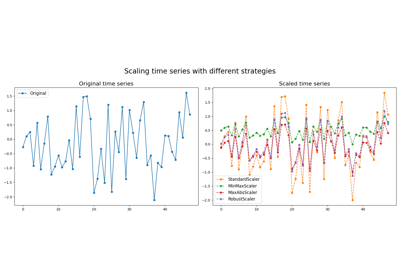# `pyts.preprocessing`.RobustScaler¶

class `pyts.preprocessing.``RobustScaler`(with_centering=True, with_scaling=True, quantile_range=(25.0, 75.0))[source]

Scale samples using statistics that are robust to outliers.

This Scaler removes the median and scales the data according to the quantile range (defaults to IQR: Interquartile Range). The IQR is the range between the 1st quartile (25th quantile) and the 3rd quartile (75th quantile).

Centering and scaling happen independently on each sample by computing the relevant statistics on the timestamps. Median and interquartile range are used to transform the data.

Standardization of a dataset is a common requirement for many machine learning estimators. Typically this is done by removing the mean and scaling to unit variance. However, outliers can often influence the sample mean / variance in a negative way. In such cases, the median and the interquartile range often give better results.

Parameters: with_centering : bool (default = True) If True, center the data before scaling. with_scaling : bool (default = True) If True, scale the data to interquartile range. quantile_range : tuple (q_min, q_max), 0.0 < q_min < q_max < 100.0 Default: (25.0, 75.0) = (1st quantile, 3rd quantile) = IQR

Examples

```>>> from pyts.preprocessing import RobustScaler
>>> X = [[1, -2,  4],
...      [-2,  1,  1],
...      [2,  3, -2]]
>>> scaler = RobustScaler()
>>> scaler.transform(X)
array([[ 0. , -1. ,  1. ],
[-2. ,  0. ,  0. ],
[ 0. ,  0.4, -1.6]])
```

Methods

 `__init__`([with_centering, with_scaling, …]) Initialize self. `fit`([X, y]) Pass. `fit_transform`(X[, y]) Fit to data, then transform it. `get_params`([deep]) Get parameters for this estimator. `set_output`(*[, transform]) Set output container. `set_params`(**params) Set the parameters of this estimator. `transform`(X) Scale the data.
`__init__`(with_centering=True, with_scaling=True, quantile_range=(25.0, 75.0))[source]

Initialize self. See help(type(self)) for accurate signature.

`fit`(X=None, y=None)[source]

Pass.

Parameters: X Ignored y Ignored self : object
`fit_transform`(X, y=None, **fit_params)

Fit to data, then transform it.

Fits transformer to X and y with optional parameters fit_params and returns a transformed version of X.

Parameters: X : array-like of shape (n_samples, n_features) Input samples. y : array-like of shape (n_samples,) or (n_samples, n_outputs), default=None Target values (None for unsupervised transformations). **fit_params : dict Additional fit parameters. X_new : ndarray array of shape (n_samples, n_features_new) Transformed array.
`get_params`(deep=True)

Get parameters for this estimator.

Parameters: deep : bool, default=True If True, will return the parameters for this estimator and contained subobjects that are estimators. params : dict Parameter names mapped to their values.
`set_output`(*, transform=None)

Set output container.

See Introducing the set_output API for an example on how to use the API.

Parameters: transform : {“default”, “pandas”}, default=None Configure output of transform and fit_transform. “default”: Default output format of a transformer “pandas”: DataFrame output None: Transform configuration is unchanged self : estimator instance Estimator instance.
`set_params`(**params)

Set the parameters of this estimator.

The method works on simple estimators as well as on nested objects (such as `Pipeline`). The latter have parameters of the form `<component>__<parameter>` so that it’s possible to update each component of a nested object.

Parameters: **params : dict Estimator parameters. self : estimator instance Estimator instance.
`transform`(X)[source]

Scale the data.

Parameters: X : array-like, shape = (n_samples, n_timestamps) Data to scale. X_new : array-like, shape = (n_samples, n_timestamps) Scaled data.

## Examples using `pyts.preprocessing.RobustScaler`¶Scalers

Scalers